# 3.5 DYSON Equation

The DYSON equation can be achieved by classifying the various contributions in arbitrary FEYNMAN diagrams. DYSON's equation summarizes the FEYNMAN-DYSON perturbation theory in a particularly compact form. The exact GREEN's function can be written as the non-interacting GREEN's function plus all connected terms with a non-interacting GREEN's function at each end, see (3.40). This structure is shown in Fig. 3.5, where the double line denotes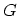and the single line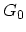.
Figure 3.5: The GREEN's function expanded in terms of connected diagrams.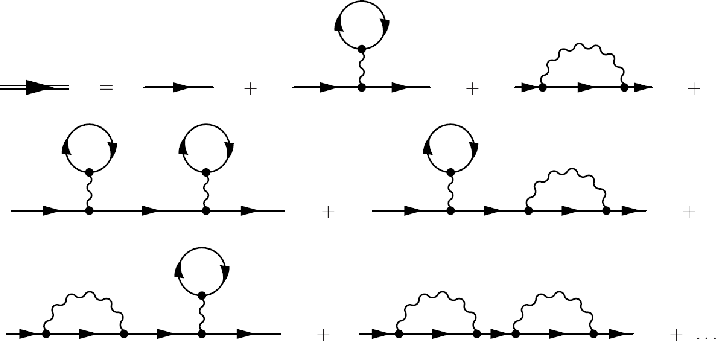By introducing the concept of self-energy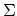the structure in Fig. 3.5 takes the form shown Fig. 3.6.

Figure 3.6: FEYNMAN diagrams showing the general structure of.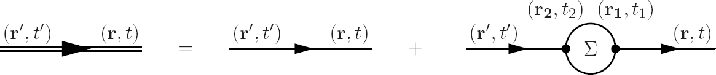The corresponding analytic expression is given by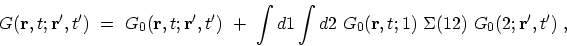(3.41)

where the abbreviationand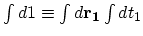is used. The self-energydescribes the renormalization of single-particle states due to the interaction with the surrounding many-particle system and the DYSON equation determines the renormalized GREEN's function.

Another important concept is the proper self-energy insertion which is a self-energy insertion that can not be separated into two pieces by cutting a single-particle line. By definition, the proper self-energy is the sum of all proper self-energy insertions, and will be denoted by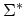. Using the perturbation expansion, one can define the proper self-energyas an irreducible part of the GREEN's function. Based on this definition first-order proper self-energies, which are resulted from the first-order expansion of the GREEN's function (see Section 3.4.2), are shown in Fig. 3.7. These diagrams are irreducible parts of Fig. 3.4-b and Fig. 3.4-c and are referred to as the HARTREE (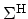) and the FOCK (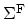) self-energies.

Figure 3.7: FEYNMAN diagrams of the first-order proper self-energies.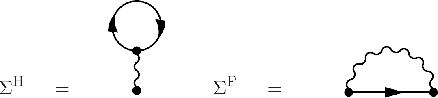The self-energy can also in principle be introduced variationally . A variational derivation of the self-energies for the electron-electron and electron-phonon interactions is presented in Appendices F.1 and F.2, respectively. It follows from these definitions that the self-energy consists of a sum of all possible repetitions of the proper self-energy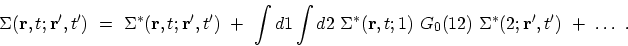(3.42)

Correspondingly, the GREEN's function in (3.41) can be rewritten as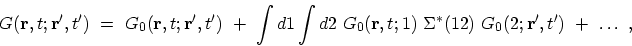(3.43)

which can be summed formally to yield an integral equation (DYSON equation) for the exact GREEN's function which is shown in Fig. 3.8.
Figure 3.8: FEYNMAN diagrams representing DYSON's equation.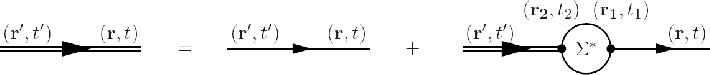The corresponding analytic expression is given by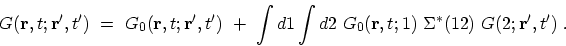(3.44)

The validity of (3.44) can be verified by iterating the right hand-side, which reproduces (3.43) term by term. In a similar manner, one can show that the DYSON equation can be also written as(3.45)

M. Pourfath: Numerical Study of Quantum Transport in Carbon Nanotube-Based Transistors